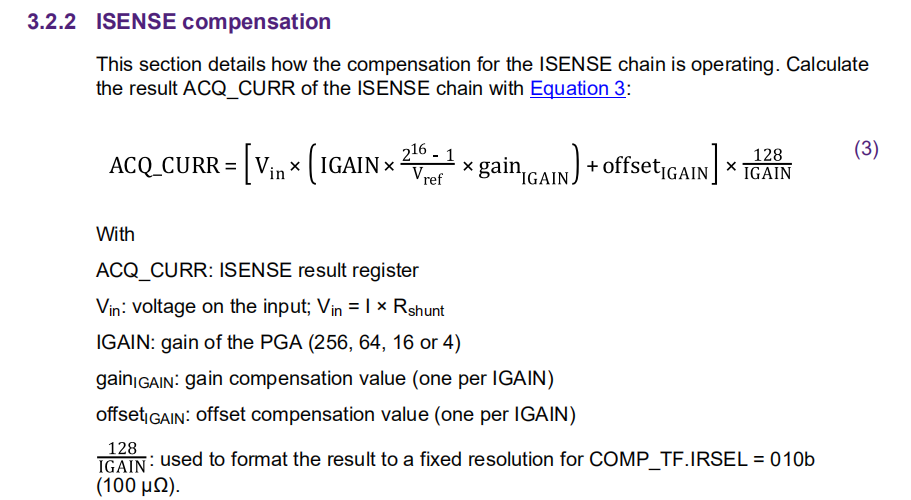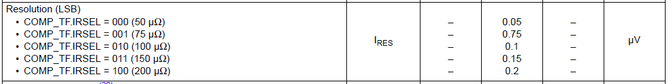# Vref of CSENSE in MM9Z1_638

cancel
Showing results for
Did you mean:
SOLVED

## Vref of CSENSE in MM9Z1_638

596 ViewsContributor I

Hi,

i tried to calculate the Vin of CSENSE block with the equation in AN12301(MM9Z1X638 system calibration guidelines) as follows:but i cant find Vref of CSENSE in datasheet. Is this the right way to calculate the measured current? And please let me know how to get the correct current if my Shunt Resistor is not 100 uOhm.

THANKS!

Labels (1)
• ### Battery Sensors

1 Solution
587 ViewsNXP TechSupport

Hi Xu Shaojun,

DESCRIPTION

The Vref is 1.25V. We do not specify it as its actually not required for any customer calculations (except GCB).

The resolution of the ISENSE is fixed, but a parameter of the selected shunt value IRSEL:To calculate the current measured:

e.g. for COMP_TF-IRSEL = 0b010 -> LSB = 0.1uV   (”IRES” from table above)

U_measured = Ushunt = LSB * ADC_Value

I_measured = LSB * ADC_value *  1 / Rshunt

You can calculate the current-LSB =  LSB  *  1/ Rshunt

e.g.

for 100uOhm:     0.1uV  *  1/100 uV/A  = 1mA

for 50uOhm:       0.1uV  *  1/50 uV/A  = 2mA

If you use a different COM_TF.IRSEL the LSB will change. However I recommend to use the 100uOhm configuration even if the shunt value is not 100uOhm. Current measurement result is not clamping for shunt resistor selection 50 and 75.

With Best Regards,

Jozef

588 ViewsNXP TechSupport

Hi Xu Shaojun,

DESCRIPTION

The Vref is 1.25V. We do not specify it as its actually not required for any customer calculations (except GCB).

The resolution of the ISENSE is fixed, but a parameter of the selected shunt value IRSEL:To calculate the current measured:

e.g. for COMP_TF-IRSEL = 0b010 -> LSB = 0.1uV   (”IRES” from table above)

U_measured = Ushunt = LSB * ADC_Value

I_measured = LSB * ADC_value *  1 / Rshunt

You can calculate the current-LSB =  LSB  *  1/ Rshunt

e.g.

for 100uOhm:     0.1uV  *  1/100 uV/A  = 1mA

for 50uOhm:       0.1uV  *  1/50 uV/A  = 2mA

If you use a different COM_TF.IRSEL the LSB will change. However I recommend to use the 100uOhm configuration even if the shunt value is not 100uOhm. Current measurement result is not clamping for shunt resistor selection 50 and 75.

With Best Regards,

Jozef